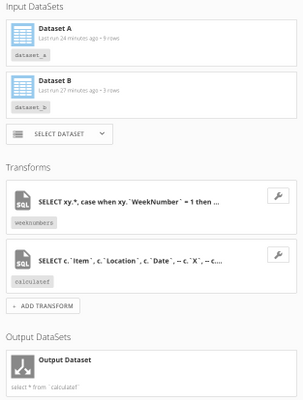# Calculating a value for current week and rolling that value for subsequent weeks

Hi,

I have the following 2 datasets:

Dataset A

`Item  Location   Date                  X    Y  A      USA     Sep 10(Current Week)  10   10  A      USA     Sep 17                15   20           A      USA     Sep 24                10    5  A     Canada   Sep 10(Current Week)   0    4  A     Canada   Sep 17                 6    0  A     Canada   Sep 24                 13   15  B      USA     Sep 10(Current Week)   10   50  B      USA     Sep 17                 5    15  B      USA     Sep 24                 15   20`

Dataset B:

`  Item    Location     Z   A        USA        15      A        Canada     0      B         USA       25`

I need an output dataset as follows:

O/P

`   Item   Location   Date   Final_Column    A      USA      Sep 10     15        A      USA      Sep 17     20        A      USA      Sep 24     15        A    Canada     Sep 10      4        A    Canada     Sep 17      0       A    Canada     Sep 24      2        B      USA      Sep 10      65        B      USA      Sep 17      75        B      USA      Sep 24      80`

The calculation is as follows:

`   For current week, Final Column = (Z - X) + Y ----> 1st week value   For 2nd week, Final Column = (1st week value - X) + Y  ----> 2nd week value   For 3rd week, Final Column = (2nd week value - X) + Y  ----> 3rd week value `

and so on ...

` Additionally, if value of Final Column < 0 then Final Column = 0.`

How to achieve this calculation in MySQL?

• Hi,

I came up with this

`select a.item, a.location, a.date,@value = case when @item <> a.item or @location <> a.location then b.z - a.x + a.yelse @value - a.x + a.y end final_column from  a inner join b on a.item = b.item and a.location = b.location,(select @value := 0, @item := '', @location := '') xorder by a.item, a.location, a.date`

I am getting the correct values except for when the value of final column becomes less than 0.

When the final_column value goes less than 0 , it should be hard-coded to 0.

How to achieve this in the code above?

Thanks.

• Hi, Prajju,

This is an interresting challenge! The part that makes it difficult is that, at each recursive step in the calculation, the final ouput must not be less than 0 (set to 0 if less than 0).

I did this in a SQL dataflow using two transforms. The first transform assigns a week order number, which is simply a row number partitioned by location and item and ordered by date. The second dataflow uses that week number as an input and calculates the final output value. I suppose it might be possible to combine those two steps into one, but this makes sense in my mind. Screen shot below.

The first transform:

`SELECT  xy.*,  case when xy.`WeekNumber` = 1 then b.Z else 0 end as Z -- Z is only important in the week 1 calculationfrom (  SELECT    a.Date,    a.X,    a.Y,    case       when @PrevLocation = a.`Location` and @PrevItem = a.`Item` then @WeekNumber := @WeekNumber + 1 -- if the current record has the same location and item as the previous record, then @WeekNumber = @WeekNumber + 1; otherwise, this must be week 1      else @WeekNumber := 1       end as WeekNumber,@PrevLocation := a.`Location` as Location,@PrevItem := a.`Item` as Item  from     `dataset_a` as a,    (select @WeekNumber := 0, @PrevLocation := '', @PrevItem := '') as init -- The From statement of the query is read first, so initialize variables here  order by a.`Location`,a.`Item`,a.`Date` -- since the variables are evaluated in the order in which they appear in the select statement, ordering is important) as xyleft join `dataset_b` as b  on b.`Item` = xy.`Item`  and b.`Location` = xy.`Location``

The second transform:

`SELECT  c.`Item`,  c.`Location`,  c.`Date`,  case     when c.`WeekNumber` = 1 then @F := c.`Z` - c.`X` + c.`Y` -- if the current record is week 1, the final value F = Z - X + Y    when c.`WeekNumber` > 1 and @F - c.`X` + c.`Y` > 0 then @F := @F - c.`X` + c.`Y` -- if the current record is week 2 or later (and if the previous value of F - current X + current Y > 0), final value is calculated recursively: F = F - X + Y    when c.`WeekNumber` > 1 and @F - c.`X` + c.`Y` <= 0 then @F := 0 -- if the current record is week 2 or later (and if the previous value of F - current X + current Y <= 0), final value is F = 0    end as final_columnfrom `weeknumbers` as c,(select @F := 0) as init -- initialize the variable Forder by c.`Item`,c.`Location` desc,c.`WeekNumber`,c.`Date` -- again, since F is calculated incrementally, order matters`

Hope this works for you.• Hi Dan,

I actually came up with a relatively easier solution:

`select a.item, a.location, a.date,@value = case when @item <> a.item or @location <> a.location then (ABS(b.z - a.x + a.y) + (b.z - a.x + a.y))/2else (ABS(@value - a.x + a.y) + (@value - a.x + a.y))/2 end final_column from a inner join b on a.item = b.item and a.location = b.location, (select @value := 0, @item := '', @location := '') xorder by a.item, a.location, a.date`

So basically, if a value is negative, code it to 0. For example, if value = -15: (ABS(-15) + (-15)) / 2 --> (15 - 15)/2 --->  0

If positive retain the value . If value = 15 : (ABS(15) + 15)/2 ---> 15

Thanks,

Prajwal